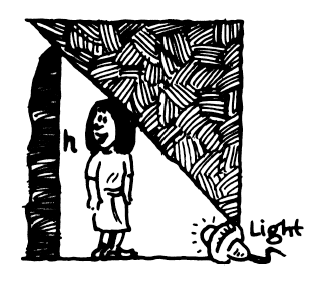### Home > PC > Chapter 6 > Lesson 6.3.2 > Problem6-128

6-128.The shadow of Kate is cast on a wall by a light on the ground, which is $d$ meters from the wall. Kate is $162$ centimeters tall and is 3 meters from the wall. Express $d$ (in meters) as a function of the height of the shadow, $h$ (in meters).

1. Label the drawing.
2. Convert Kate's height to meters.
3. You have two similar triangles. Write a proportion with $d$ and $h$.
4. Solve for $d$.

$\frac{d-3}{d}=\frac{1.62}{h}$